General Information

Below are online physics problems for First Year Mechanics, Phy131. Pick a problem and start by clicking on "New n1 & n2". After determining the answer, enter it in the text box "Input n3:", then click "Grade it". The program will display "correct" or "incorrect". If you want to try the calculation again click "New n1 & n2". All answers are integers. You should not need a calculator to solve the problems. Only type an integer as an answer. If my students want credit for answering the problem correctly, then type in your id and click "record grade". Let me know if you have any difficulties in understanding the questions.

## Phy131

### Statics: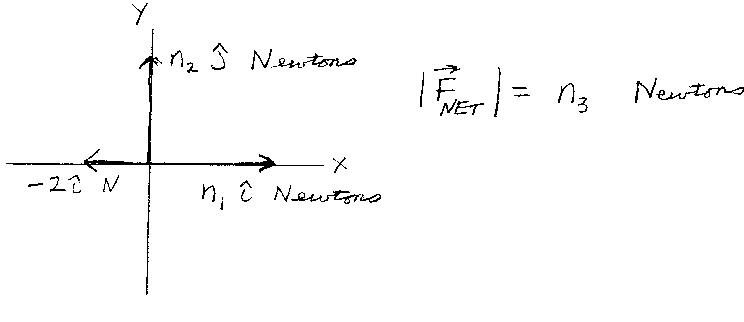Problem A1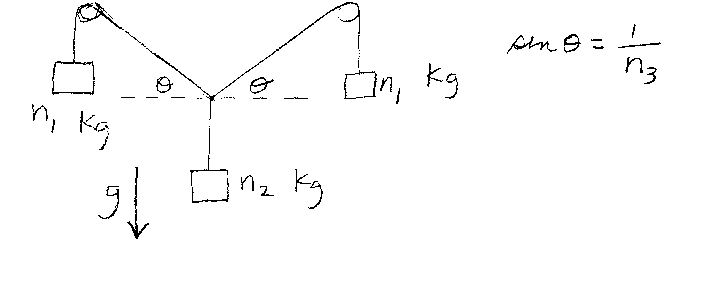Problem A2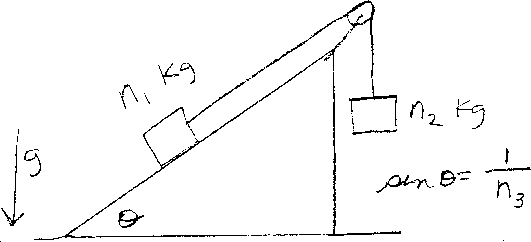Problem A3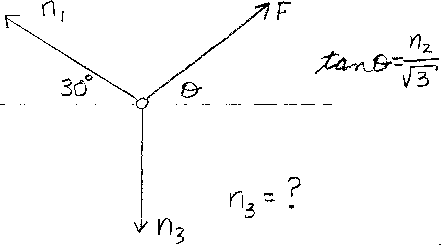Problem A4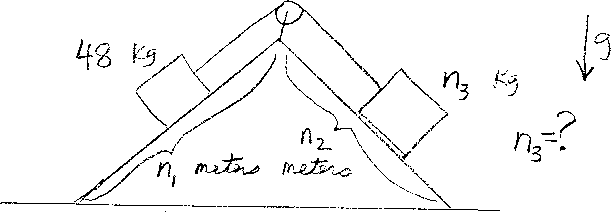Problem A5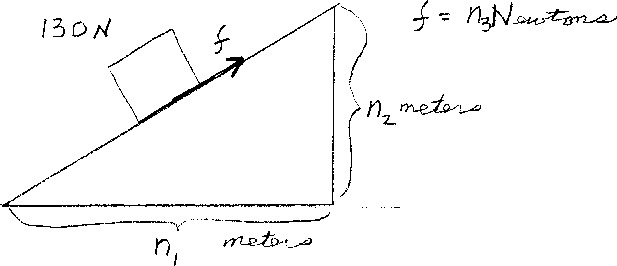Problem A6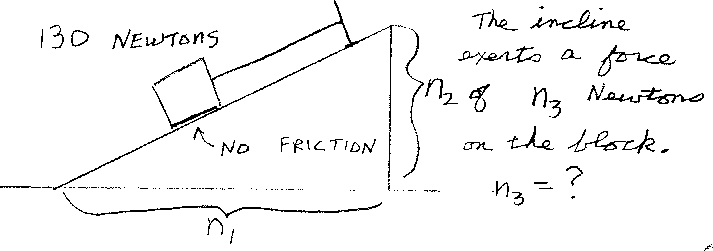Problem A7Problem A8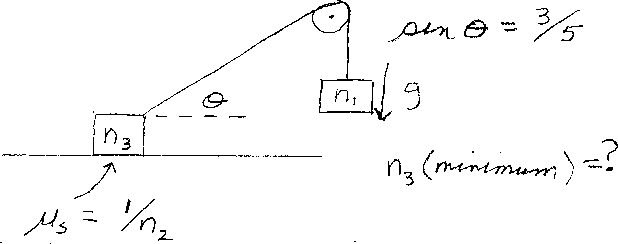Problem A9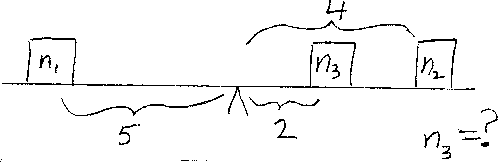Problem A10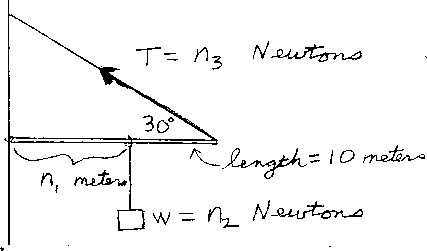Problem A11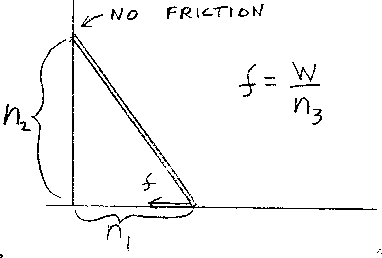Problem A12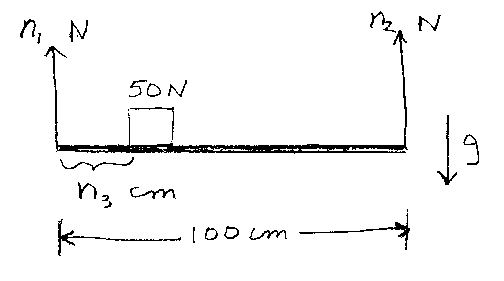Problem A13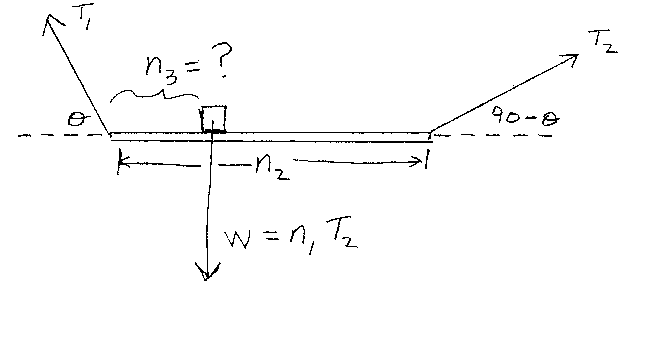Problem A14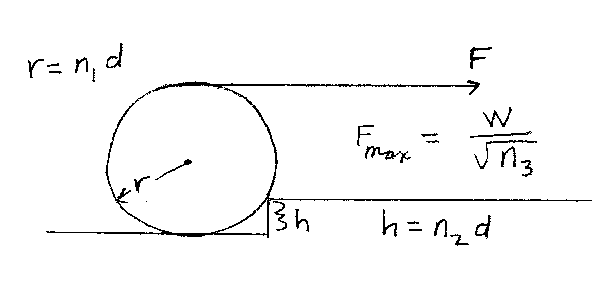Problem A15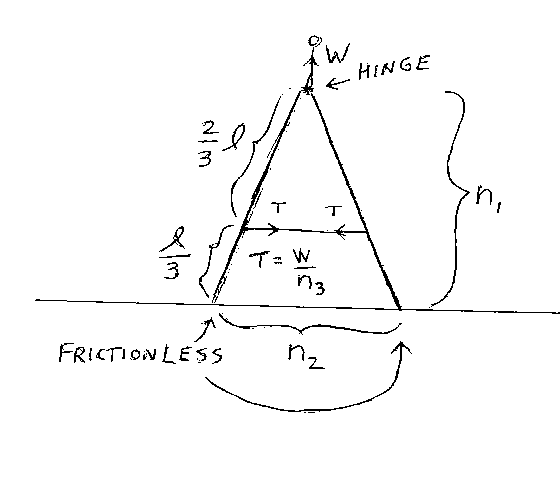Problem A16

### Force and Motion: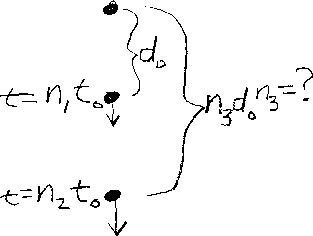Problem B1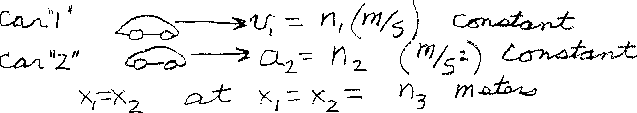Problem B2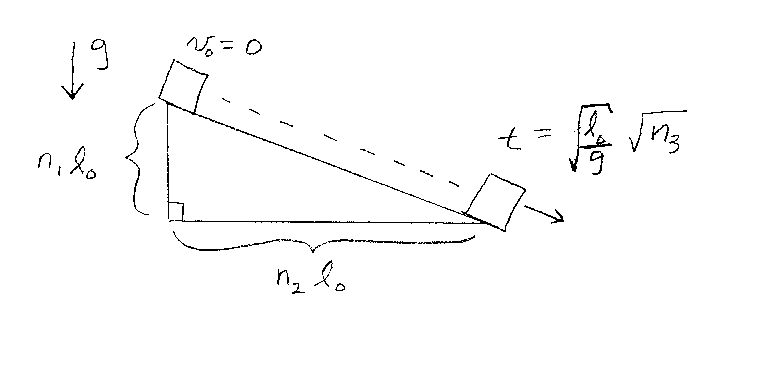Problem B3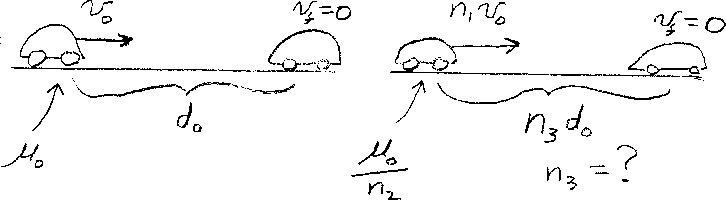Problem B4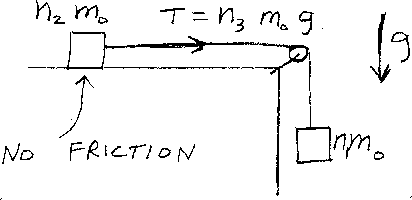Problem B5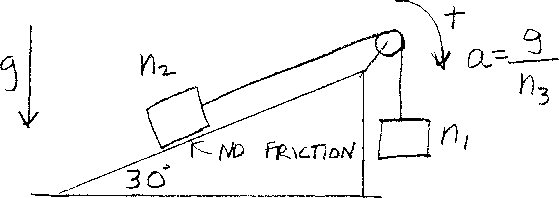Problem B6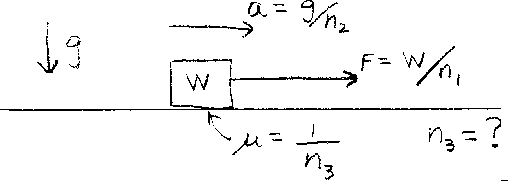Problem B7Problem B8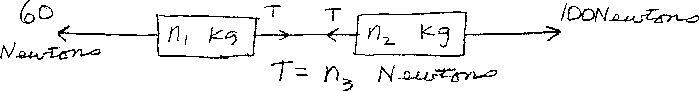Problem B9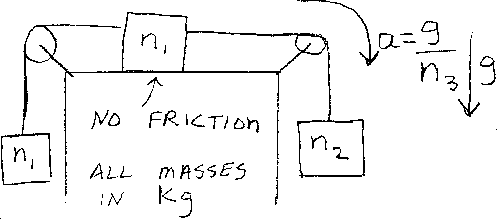Problem B10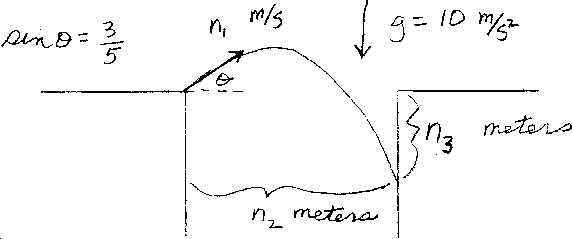Problem B11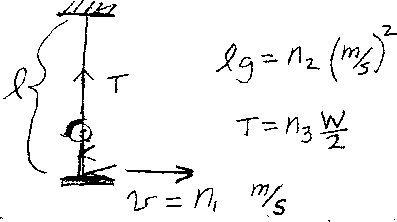Problem B12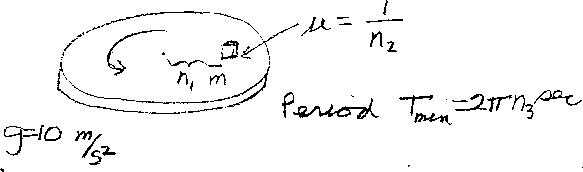Problem B13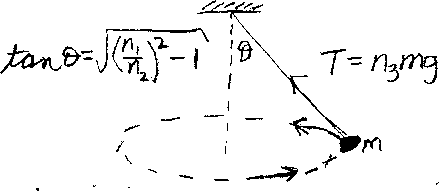Problem B14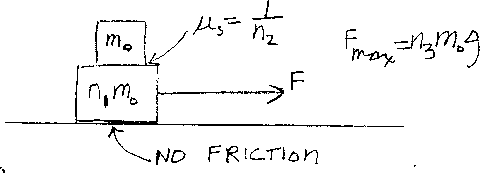Problem B15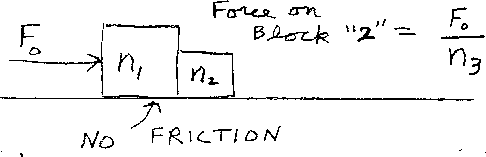Problem B16

### Momentum and Mechanical Energy: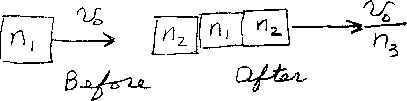Problem C1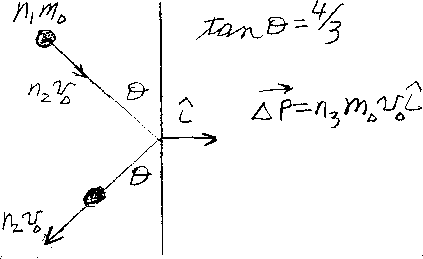Problem C2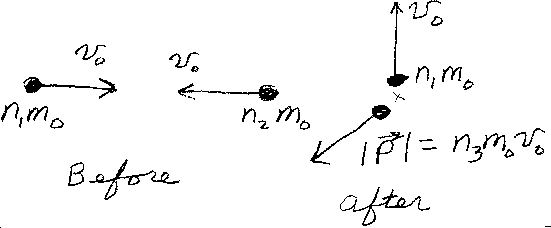Problem C3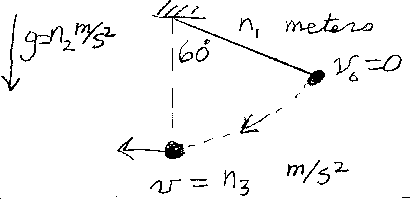Problem C4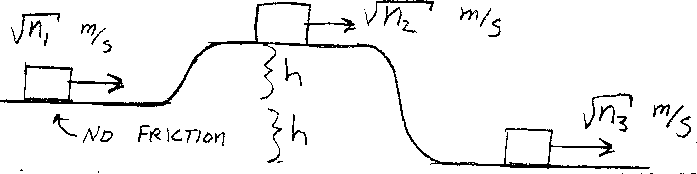Problem C5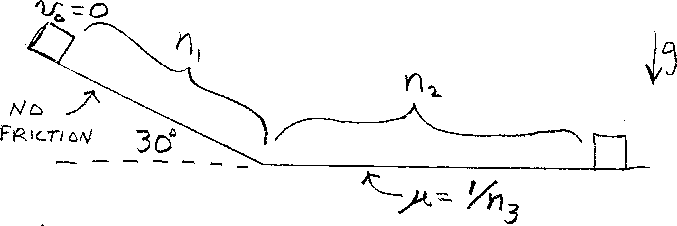Problem C6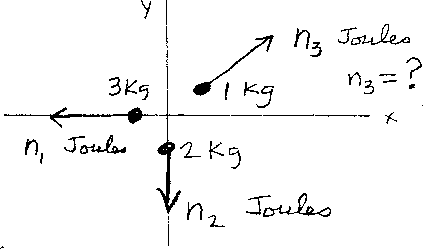Problem C7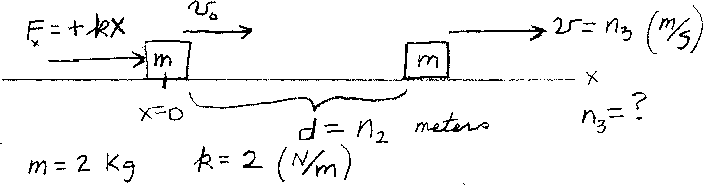Problem C8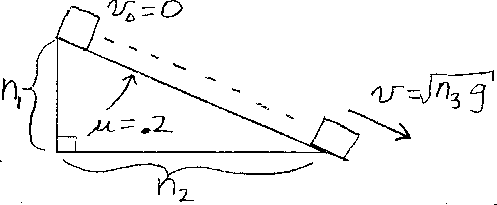Problem C9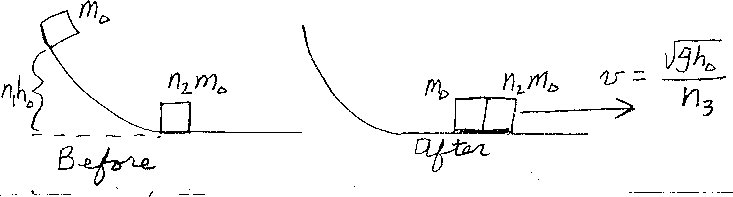Problem C10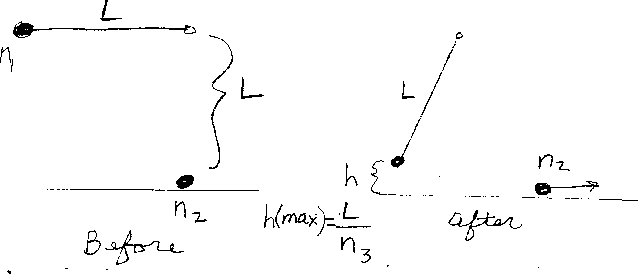Problem C11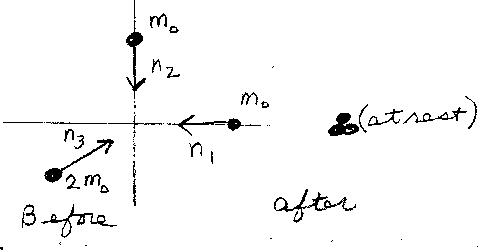Problem C12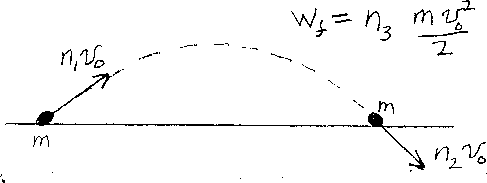Problem C13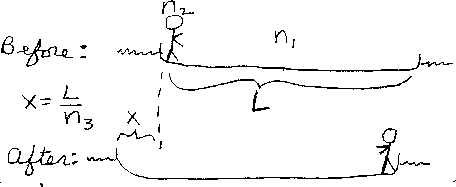Problem C14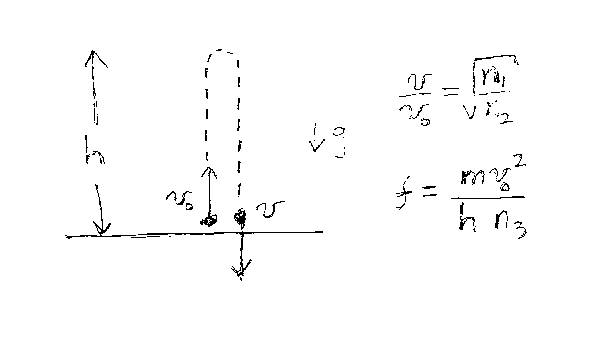Problem C15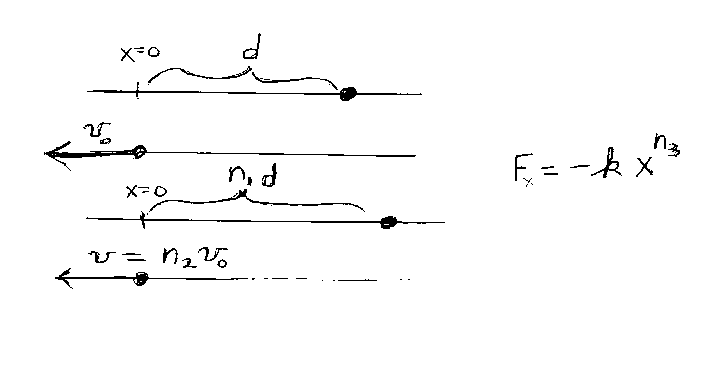Problem C16

### Rotational Dynamics: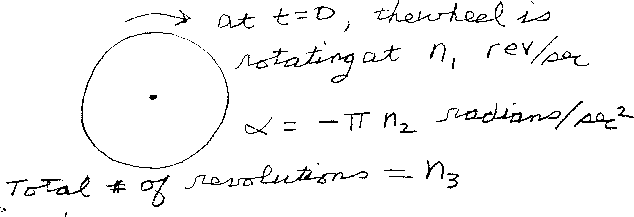Problem D1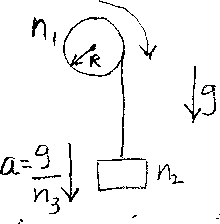Problem D2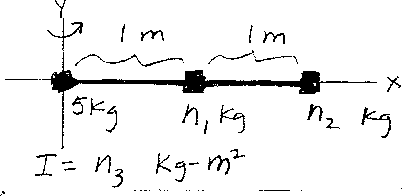Problem D3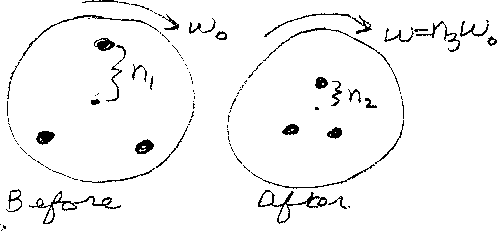Problem D4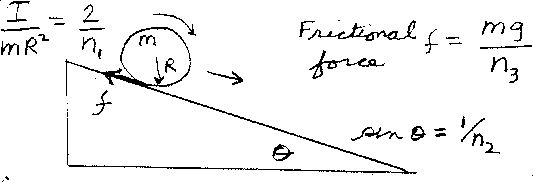Problem D5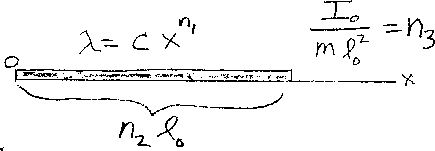Problem D6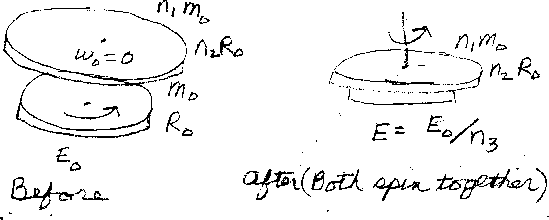Problem D7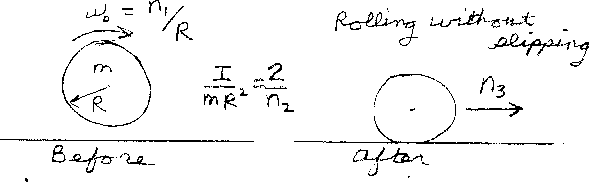Problem D8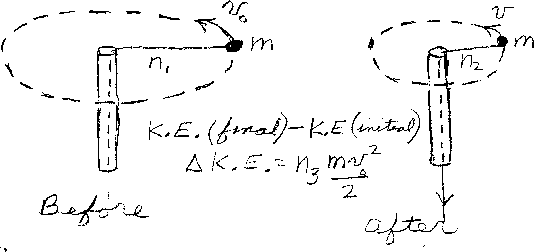Problem D9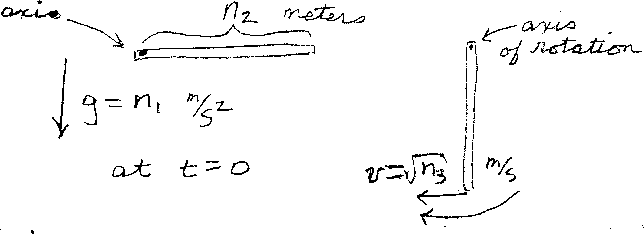Problem D10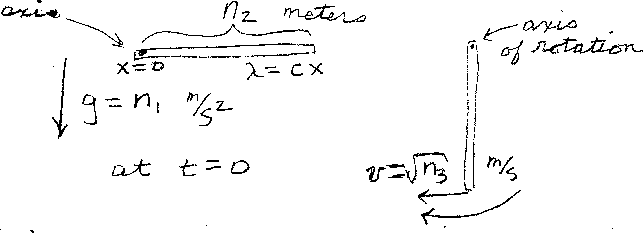Problem D11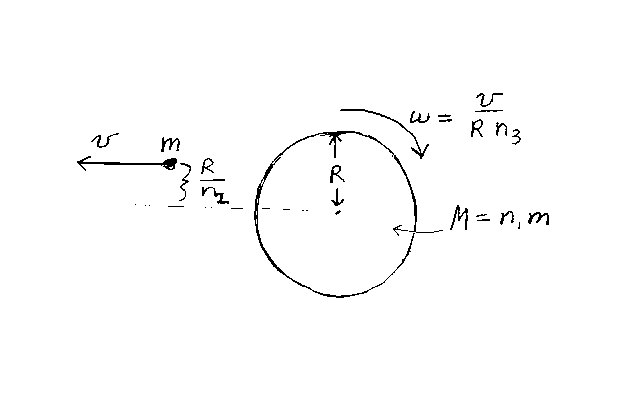Problem D12